Miscellaneous

Chapter 15 Class 11 Statistics (Term 1)
Serial order wise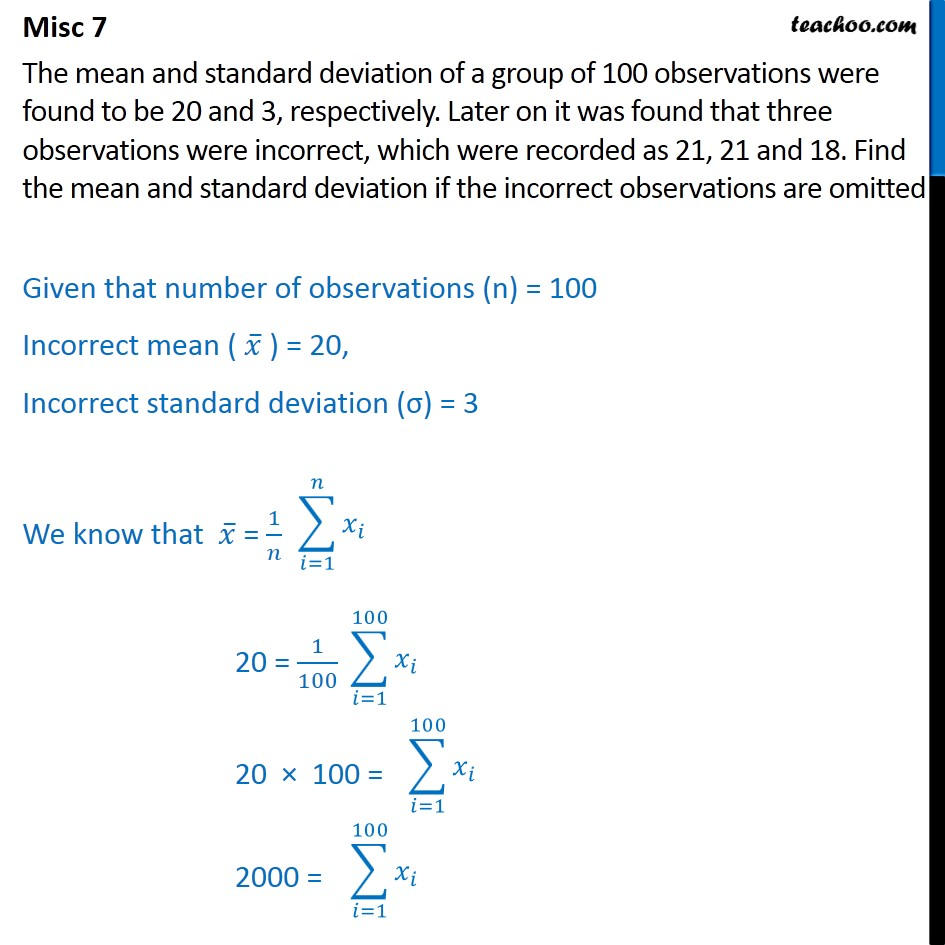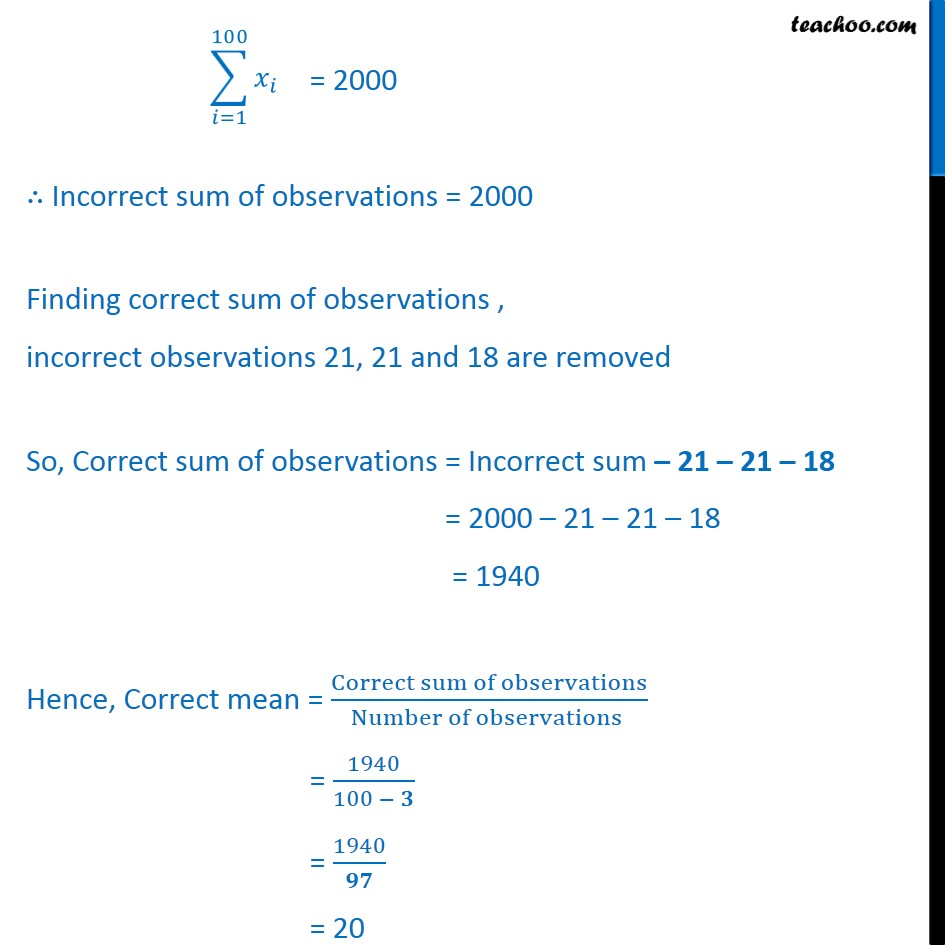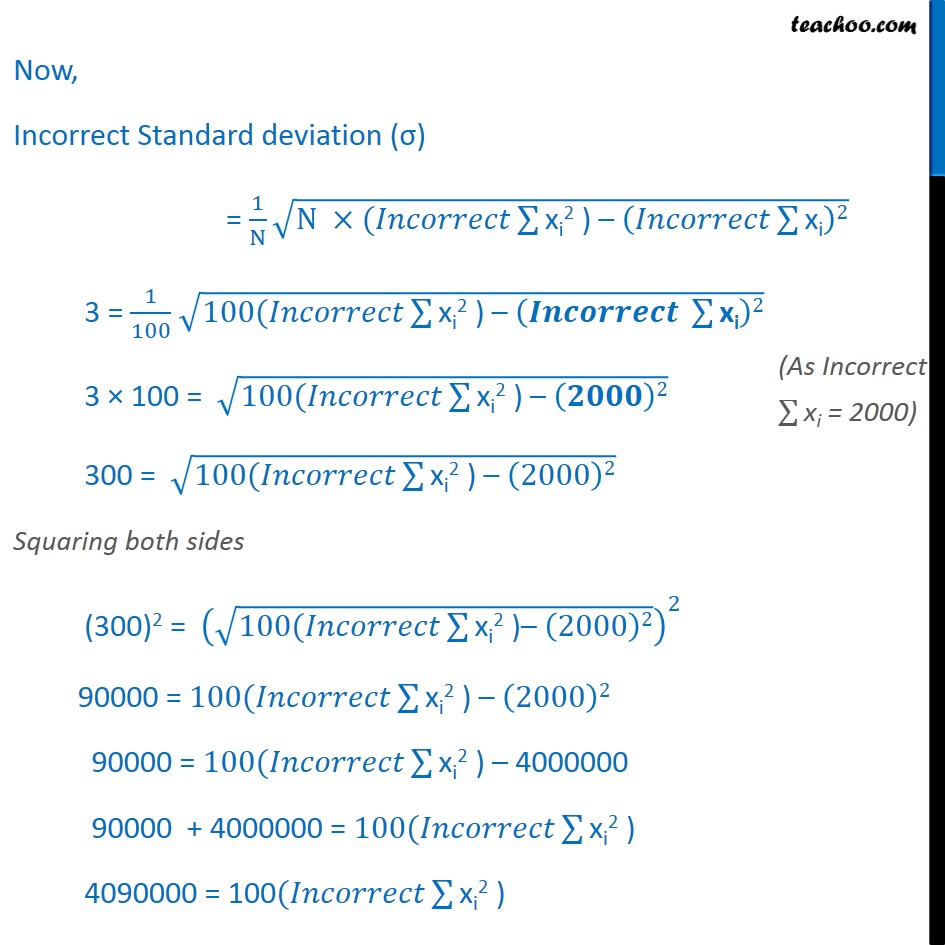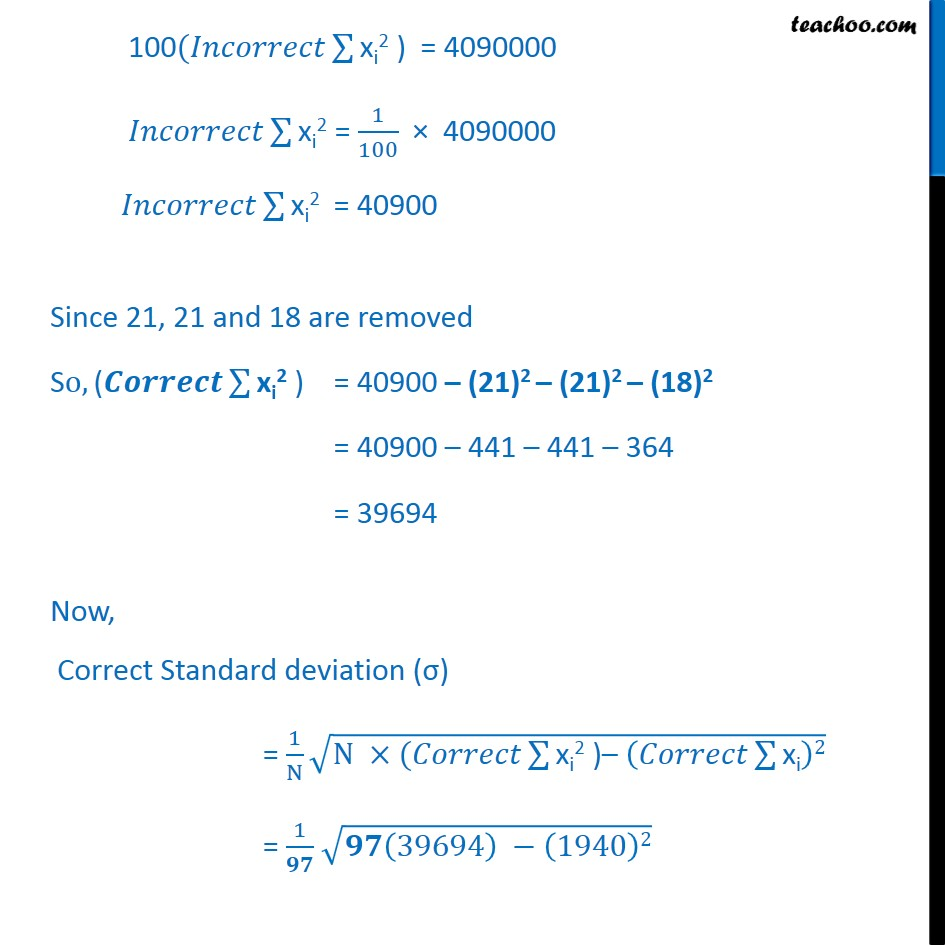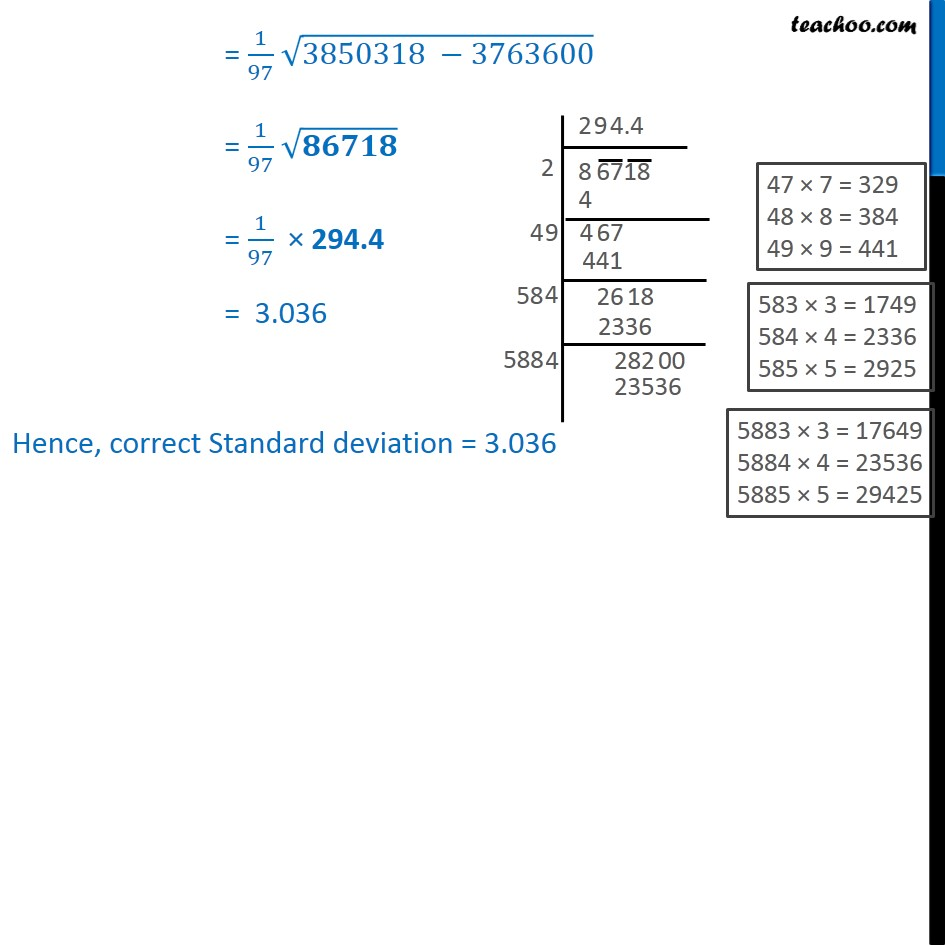### Transcript

Misc 7 The mean and standard deviation of a group of 100 observations were found to be 20 and 3, respectively. Later on it was found that three observations were incorrect, which were recorded as 21, 21 and 18. Find the mean and standard deviation if the incorrect observations are omitted Given that number of observations (n) = 100 Incorrect mean ( 𝑥﷯ ) = 20, Incorrect standard deviation (σ) = 3 We know that 𝑥﷯ = 1﷮𝑛﷯ 20 = 1﷮100﷯ 20 × 100 = 2000 = = 2000 ∴ Incorrect sum of observations = 2000 Finding correct sum of observations , incorrect observations 21, 21 and 18 are removed So, Correct sum of observations = Incorrect sum – 21 – 21 – 18 = 2000 – 21 – 21 – 18 = 1940 Hence, Correct mean = Correct sum of observations﷮Number of observations ﷯ = 1940﷮100 − 𝟑﷯ = 1940﷮𝟗𝟕﷯ = 20 Now, Incorrect Standard deviation (σ) = 1﷮N﷯ ﷮N ×(𝐼𝑛𝑐𝑜𝑟𝑟𝑒𝑐𝑡 ﷮﷮xi2 ) – 𝐼𝑛𝑐𝑜𝑟𝑟𝑒𝑐𝑡 ﷮﷮xi﷯﷯2﷯﷯ 3 = 1﷮100﷯ ﷮100(𝐼𝑛𝑐𝑜𝑟𝑟𝑒𝑐𝑡 ﷮﷮xi2 ) – 𝑰𝒏𝒄𝒐𝒓𝒓𝒆𝒄𝒕 ﷮﷮xi﷯﷯2﷯﷯ 3 × 100 = ﷮100(𝐼𝑛𝑐𝑜𝑟𝑟𝑒𝑐𝑡 ﷮﷮xi2 ) – 𝟐𝟎𝟎𝟎﷯2﷯﷯ 300 = ﷮100(𝐼𝑛𝑐𝑜𝑟𝑟𝑒𝑐𝑡 ﷮﷮xi2 ) – 2000﷯2﷯﷯ Squaring both sides (300)2 = ﷮100(𝐼𝑛𝑐𝑜𝑟𝑟𝑒𝑐𝑡 ﷮﷮xi2 )– 2000﷯2﷯﷯﷯﷮2﷯ 90000 = 100(𝐼𝑛𝑐𝑜𝑟𝑟𝑒𝑐𝑡 ﷮﷮xi2 ) – 2000﷯2﷯ 90000 = 100(𝐼𝑛𝑐𝑜𝑟𝑟𝑒𝑐𝑡 ﷮﷮xi2 ) –﷯ 4000000 90000 + 4000000 = 100(𝐼𝑛𝑐𝑜𝑟𝑟𝑒𝑐𝑡 ﷮﷮xi2 ) ﷯ 4090000 = 100(𝐼𝑛𝑐𝑜𝑟𝑟𝑒𝑐𝑡 ﷮﷮xi2 ) ﷯ 100(𝐼𝑛𝑐𝑜𝑟𝑟𝑒𝑐𝑡 ﷮﷮xi2 ) ﷯ = 4090000 𝐼𝑛𝑐𝑜𝑟𝑟𝑒𝑐𝑡 ﷮﷮xi2 ﷯= 1﷮100﷯ × 4090000 𝐼𝑛𝑐𝑜𝑟𝑟𝑒𝑐𝑡 ﷮﷮xi2 ﷯ = 40900 Since 21, 21 and 18 are removed So, (𝑪𝒐𝒓𝒓𝒆𝒄𝒕 ﷮﷮xi2 ﷯) = 40900 – (21)2 – (21)2 – (18)2 = 40900 – 441 – 441 – 364 = 39694 Now, Correct Standard deviation (σ) = 1﷮N﷯ ﷮N ×(𝐶𝑜𝑟𝑟𝑒𝑐𝑡 ﷮﷮xi2 )– 𝐶𝑜𝑟𝑟𝑒𝑐𝑡 ﷮﷮xi﷯﷯2﷯﷯ = 1﷮𝟗𝟕﷯ ﷮𝟗𝟕 39694﷯ − 1940﷯2﷯ = 1﷮97﷯ ﷮3850318 −3763600﷯ = 1﷮97﷯ ﷮𝟖𝟔𝟕𝟏𝟖﷯ = 1﷮97﷯ × 294.4 = 3.036 Hence, correct Standard deviation = 3.036